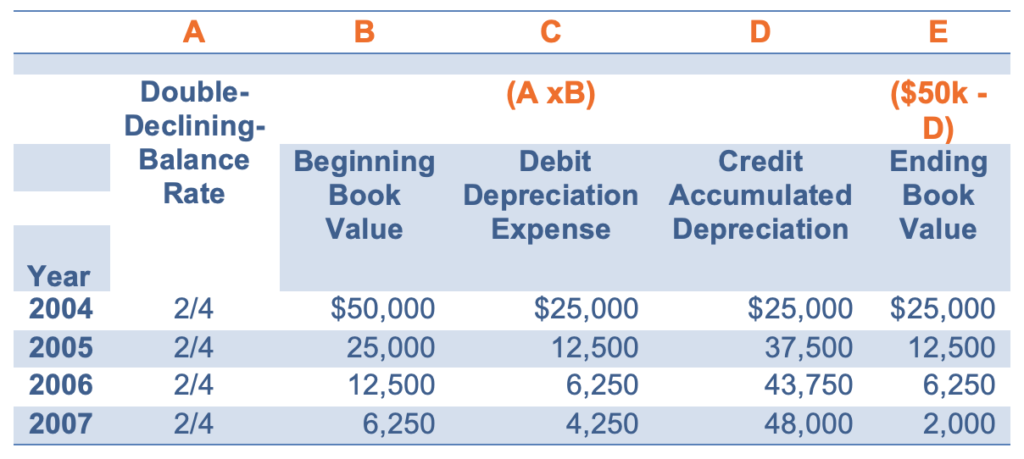# Depreciation Expense Recognition

A company’s long-lived assets refer to PPE, natural resources, and intangible assets. Since these assets provide economic value over multiple years, the cost of these assets is allocated over multiple years.

Depreciation is the allocation of the cost of an asset to expense over its useful life in a rational and systematic manner.

There are various depreciation methods used by firms. We will look at the following three methods:

• Straight Line Method
• Accelerated Methods of Depreciation

### Straight Line Method

The straight-line method associates the long-lived asset’s usefulness with its age.

Straight-Line Expense = (Cost – Salvage Value)/n

where n = number of years in asset’s useful life

Example

A food processing company purchased equipment on January 1, 2012, at a cost of $50,000. Management expects the equipment to have a four-year life and a$2,000 residual value.

The annual depreciation expense will be = (50,000 – 2,000)/4 = $12,000 ### Accelerated Methods of Depreciation Accelerated depreciation allocates a larger portion of the cost of a plant asset to expense early in the asset’s life. The accelerated methods of depreciation include Sum-of-the-Years Digits (SYD) expensing method and declining balance methods. ### SYD Method SYD method treats an asset as more useful in its early life by raising the depreciation expense for the early years. SYD Example: If a company’s factory has a new conveyor belt with a useful life of 5 years, then SYD = 1+2+3+4+5 = 15. This conveyor belt cost$100,000 and has a salvage value = $0. The year two depreciation expense under the SYD method for the company will be calculated as follows: ($100,000 – $0) * (5 – 2 +1)/15 =$100,000*(4/15) = $26,667 SYD Depreciation Expense for Year “i” = (Cost – Salvage Value) * ((n – “# of the ithyear” +1))/SYD ### Declining Balance Method Under this method, we apply a constant rate of depreciation to the book value of the asset every year. This method is also called diminishing balance method. Double-Declining-Balance (DDB) is an example of a declining balance method. The DDB method accelerates the depreciation rate of the straight line method. DDB Expense = (Cost – Accumulated Depreciation) * (2/n) If the food processing company used double-declining-balance method, it would multiply the straight-line rate by two, e.g. 2/1 x ¼ = 2/4 In the first year, the double-declining-balance depreciation expense = 50,000 * 2/4 =$25,000

In the second year, the double-declining-balance depreciation expense = (50,000 – 25,000) * 2/4 = \$12,500.

The depreciation schedule will be as follows:Note that in the 4th year, the depreciation expense is equal to Beginning Book Value – Residual Value = 6,250 – 2,000 = 4,250.

Reasons for using Accelerated Depreciation

• An asset is more useful earlier in its life than later, and the useful life may be difficult to estimate.
• Depreciation expense is deductible in computing taxable income and income taxes.